# 登楼山隧道通风方案比选研究Study on Comparison and Selection of Ventilation Schemes for Dengloushan Tunnel

• 全文下载: PDF(1333KB)    PP.772-783   DOI: 10.12677/HJCE.2019.83090
• 下载量: 61  浏览量: 140

In order to explore the ventilation scheme of the G8012 Mile-Chuxiong highway project from the Mile to Yuxi section of the Dengloushan tunnel, based on the calculation of the required air volume of the Dengloushan tunnel, considering the near and long-term planning of the left and right tunnels and adding to the practical construction conditions, the paper proposes two ventilation schemes that are “two segments in the left line of three-inclined well, three segments with longitudinal ventilation of the right line” and the “two segments in the left line of three-shaft, three segments with longitudinal ventilation of the right line”. The comparison results show that: three-shaft well’s ventilation shaft length is below 500 m, the three-inclined shaft scheme is about 1500 m, and the ventilation resistance of the three-inclined well is large, resulting in the fan installed power increasing 1654 kW, the growth ratio is 15.3%, and the operation energy consumption and the civil engineering quantity increase, which is not suitable. It is recommended the “two segments in the left line of three-inclined well, three segments with longitudinal ventilation of the right line” as the ventilation scheme of the tunnel, and the 1250 type large thrust jet fan should be selected to replenish the pressurization.

1. 引言

2. 登楼山隧道概况Table 1. Main design parameters of Dengloushan tunnel

3. 登楼山隧道通风方案及比选

3.1. 隧道需风量的计算Table 2. Calculation results of required air volume under different working conditions of Dengloushan tunnel

3.2. 登楼山隧道通风方案及比选

3.2.1. 方案一：三斜井，左线两段，右线分三段纵向通风

1) 通风分段划分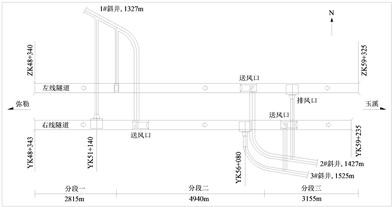Figure 1. Schematic diagram of three inclined well ventilation scheme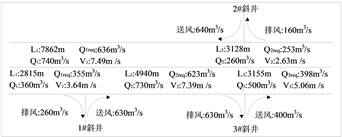Figure 2. Long-term air volume layout of three inclined well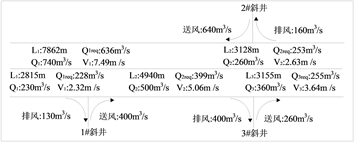Figure 3. Recent air volume layout of the three inclined well

1#斜井排风道及2#斜井的排风道需要兼顾火灾工况下的排烟需求，排烟量需大于300 m3/s。由于并联设置的轴流风机性能参数和型号应一致，为兼顾运营通风和火灾排烟，需增设一台同型号轴流风机用于火灾排烟。即1#斜井排风道设置3台排风量为130 m3/s的轴流风机，正常运营开启2台，设计风量260 m3/s，火灾工况开启3台，排烟量390 m3/s；2#斜井排风道设置2台排风量为160 m3/s的轴流风机，正常运营开启1台，设计风量160 m3/s，火灾工况开启2台，排烟量320 m3/s。远期风量及排烟量布置情况如图4所示。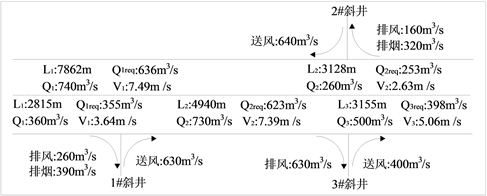Figure 4. Long-term air volume and smoke exhaust layout of three inclined well

2) 通风井断面设置Table 3. Each section’s area of three inclined well ventilation scheme

3) 通风计算

a) 送排风压力

$\Delta {P}_{e}$ ——排风口升压力(N/m2)；

$\Delta {P}_{b}$ ——送风口升压力(N/m2)；

${Q}_{r1}$ ——第I区段设计风量(m3/s)；

${V}_{r1}$ ——第I区段设计风速， ${V}_{r1}=\frac{{Q}_{r1}}{{A}_{r}}$

${Q}_{r2}$ ——第II区段设计风量(m3/s)；

${V}_{r2}$ ——第II区段设计风速；

${Q}_{e}$ ——排风量(m3/s)；

${V}_{e}$ ——与Qe相应的排风口风速；

$\alpha$ ——排风口喷流方向与隧道方向夹角，本项目排风口夹角为90˚；

$\beta$ ——送风口喷流方向与隧道方向夹角，本项目送风口夹角为0˚。

b) 气流浓度设计判定

${C}_{2}=\frac{{Q}_{req1}}{{Q}_{r1}}$ (3)

${C}_{3}=\frac{{Q}_{req2}}{{Q}_{r1}-{Q}_{e}-{Q}_{req1}+\frac{{Q}_{e}\cdot {Q}_{req1}}{{Q}_{r1}}+{Q}_{b}}$ (4)

$\frac{{Q}_{e}}{{Q}_{r1}}\le 1.0$

c) 设计浓度应满足下列条件

$0.9\le {C}_{2}\le 1.0$

$0.9\le {C}_{3}\le 1.0$

d) 所需压力设计判定

$\Delta P=\Delta {P}_{r}-\Delta {P}_{t}+\Delta {P}_{m}=\left(\Delta {P}_{r\text{I}}+\Delta {P}_{r\text{II}}\right)-\left(\Delta {P}_{t\text{I}}+\Delta {P}_{t\text{II}}\right)+\Delta {P}_{m}$ (5)

$\Delta P$ ——隧道内所需压力增量N/m2

$\Delta {P}_{r}$ ——隧道内沿程磨阻损失N/m2；对于I段，没有隧道出口流体压力损失，对于II段，没有隧道入口流体压力损失，但均应考虑竖井分叉损失，按照规范取值；

$\Delta {P}_{t}$ ——汽车交通活塞作用升压力N/m2

$\Delta {P}_{m}$ ——自然风阻抗力N/m2

ζ入口 = 0.5；ζ分歧 = 0.28；ζ出口 = 1；ζ合流 = 0.7。

e) 计算结果Table 4. Ventilation system scale of three inclined well scheme

3.2.2. 方案二：三竖井，左线两段，右线分三段纵向通风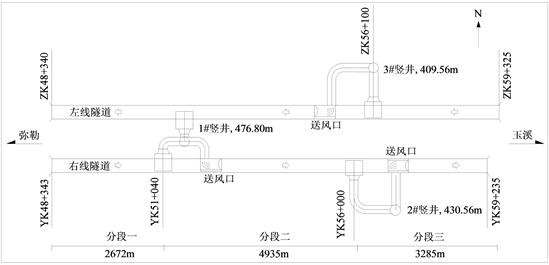Figure 5. Schematic diagram of three shaft ventilation schemeFigure 6. Long-term air volume layout of three shaft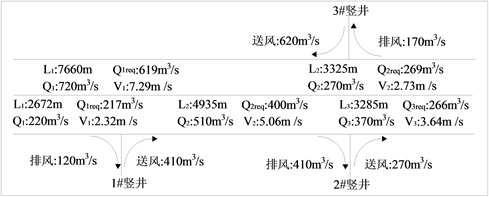Figure 7. Recent air volume layout of the three shaft

1#竖井排风道及3#竖井的排风道需要兼顾火灾工况下的排烟需求，排烟量需大于300 m3/s。由于并联设置的轴流风机性能参数和型号应一致，为兼顾运营通风和火灾排烟，需增设一台同型号轴流风机用于火灾排烟。即1#竖井排风道设置3台排风量为120 m3/s的轴流风机，正常运营开启2台，设计风量240 m3/s，火灾工况开启3台，排烟量360 m3/s；3#竖井排风道设置2台排风量为170 m3/s的轴流风机，正常运营开启1台，设计风量170 m3/s，火灾工况开启2台，排烟量340 m3/s。远期风量及排烟量布置情况如图8所示。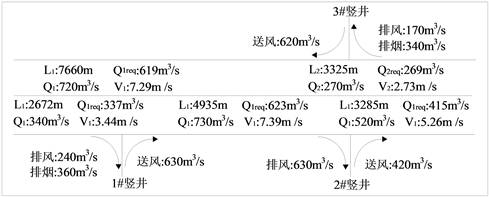Figure 8. Long-term air volume and smoke exhaust layout of the three shaft

1) 通风井断面设置Table 5. Each section’s area of three shaft ventilation scheme

2) 通风计算Table 6. Ventilation system scale of three shaft solution

3.2.3. 方案比选Table 7. Comparison and selection of ventilation schemes for Dengloushan tunnel

4. 结论

1) 根据计算，登楼山隧道左洞通风系统近期和远期控制需风量为889.76 m3/s，控制工况主要为换气。右洞近期控制需风量为883.28 m3/s，控制工况主要为换气，远期控制需风量为1377.42 m3/s，控制工况主要为稀释烟雾。

2) 登楼山隧道通风阻力较大，通风系统需设置射流风机进行补充增压，隧道断面较大可选用1250型的大推力射流风机。考虑不利情况，选取车速50 km/h进行交通力计算。

3) 三竖井方案通风井长度在500 m以下，三斜井方案通风井长度在1500 m左右。相比于三竖井，三斜井通风距离较长，通风阻力较大，风机装机功率增大1654 kW，增长比率15.3%，运营能耗增加，土建工程量增多，不宜采用。所以，本项目通风方案推荐“三竖井左线两段，右线分三段纵向通风”。

NOTES

*第一作者。

#通讯作者。

  陈光明, 韦薇, 胡益华. 特长公路隧道斜井、竖井设计技术与经验[J]. 隧道建设, 2015, 35(4): 342-349.  夏令旭. 我国长大公路隧道通风中的几个问题[J]. 公路, 2003(5): 146-149.  崔德振, 邢金城, 凌继红, 等. 城市道路隧道通风井形式对通风效果的影响[J]. 现代隧道技术, 2014, 51(6): 136-141.  闫治国, 杨其新, 王明华, 等. 火灾工况下公路隧道竖井通风模式试验研究[J]. 土木工程学报, 2006, 39(11): 101-106.  夏永旭, 王永东, 赵峰. 秦岭终南山公路隧道通风方案探讨[J]. 长安大学学报(自然科学版), 2002, 22(5): 48-50.  王小敏, 方勇. 南大梁高速公路华蓥山特长隧道施工通风方案[J]. 现代隧道技术, 2013, 50(4): 188-196.  王明年, 李琦, 于丽, 等. 高海拔隧道通风、供氧、防灾与节能技术的发展[J]. 隧道建设, 2017, 37(10): 1209-1216.  陈璋, 陈光明, 程久胜, 等. 龙潭隧道斜井、竖井设计[J]. 公路, 2005(8): 170-175.  朱春, 张旭. 公路隧道通风设计需风量几个问题的研究[J]. 地下空间与工程学报, 2009, 5(2): 364-367.  李英, 韩常领. 法国公路隧道通风量计算方法[J]. 中外公路, 2009, 29(4): 380-383.